Definitions of Square Dance Calls and Concepts
Exchange The Triangle [C3A]

Index -->  Plus  |  A1  |  A2  |  C1  |  C2  |  C3A  |  C3B  |  C4  |  NOL  |
Definitions (Text Only) -->  Plus  |  A1  |  A2  |  C1  |  C2  |  C3A  |  C3B  |  C4  |  NOL  |
 Find call:

 \$B8@8l(BFrom Twin Triangles. EN: 10
Twin Triangles (1 \$BBP\$N(B Triangle) \$B\$+\$i!%(B JP: 10

Do a total of three Triangle Circulates except that when you become 1) the Apex dancer (for Apex-to-Apex Triangles), or 2) the In-Facing Base dancer (for Base-to-Base Triangles); do your next Circulate to the other Triangle. (From Apex-to-Apex Triangles, the Circulate is an Interlocked Triangle Circulate; from Base-to-Base Triangles, the Circulate is to the diagonal opposite position.) Exchange The Triangle can be fractionalized into thirds. EN: 20
\$B\$N(B Triangle \$B\$X(B Circulate \$B\$7\$^\$9!%(B (Apex-to-Apex Triangles \$B\$+\$i\$N(B Circulate \$B\$O(B Interlocked Triangle Circulate \$B\$H\$J\$j(B, Base-to-Base Triangles \$B\$+\$i\$N(B Circulate \$B\$OExchange The Triangle \$B\$O(B 3 \$B\$D\$N%Q!<%H\$KJ,\$1\$i\$l\$^\$9!%(B JP: 20

From Apex-to-Apex Wave-Based Triangles (Very Centers lead) EN: 30
Apex-to-Apex Wave-Based Triangles \$B\$+\$i(B (Very Centers \$B\$,%j!<%I(B)JP: 30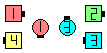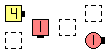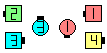Exchange The Triangles\$B\$NA0(B the left Triangle after 1/3... EN: 40 \$B:8\$N(B Triangle 1/3 \$B\$N8e(B... JP: 40 \$B8e(B
From Base-to-Base Tandem-Based Triangles (Trailing Centers lead) EN: 50
Base-to-Base Tandem-Based Triangles \$B\$+\$i(B (Trailing Centers \$B\$,%j!<%I(B)JP: 50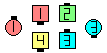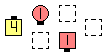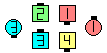Exchange The Triangles\$B\$NA0(B the left Triangle after 1/3... EN: 60 \$B:8\$N(B Triangle 1/3 \$B\$N8e(B... JP: 60 \$B8e(B

Note: As with all Exchanges, do Circulates staying on the inside of your formation until the crossover point, and then do Circulates staying on the outside of the other formation (and adjust to end on footprints of the original formation). EN: 70
\$BCm\$N(B formation \$B\$N30B&\$r(B Circulate \$B\$7\$^\$9!%\$=\$7\$F%*%j%8%J%k\$N(B formation \$B\$N(B footprint (\$BLuJP: 70

Exchange The 3 By 1 Triangle [NOL]
(Very Centers \$B\$,%j!<%I(B) JP: 80
(do a total of 4 Circulates). EN: 85
(Ciculate \$B\$r9g7W(B 4 \$B2s9T\$\$\$^\$9(B). JP: 85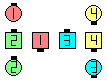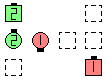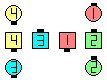Exchange The 3 By 1 Triangles\$B\$NA0(B the left Triangle after 1/4... EN: 90 \$B:8\$N(B Triangle 1/4 \$B\$N8e(B... JP: 90 \$B8e(B
Exchange The 3 By 1 Triangles [NOL]
(Very Centers \$B\$,%j!<%I(B) JP: 100
(do a total of 4 Circulates). EN: 105
(Ciculate \$B\$r9g7W(B 4 \$B2s9T\$\$\$^\$9(B). JP: 105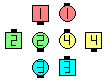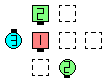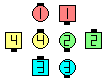Exchange The 3 By 1 Triangles\$B\$NA0(B the left Triangle after 1/4... EN: 110 \$B:8\$N(B Triangle 1/4 \$B\$N8e(B... JP: 110 \$B8e(B

\$BExchange The Box [C3A].CALLERLAB definition for Exchange The TriangleChoreography for Exchange The TriangleComments? Questions? Suggestions?

https://www.ceder.net/def/exchtriangle.php?level=C3A&language=japan&action=edit
19-January-2020 10:31:47